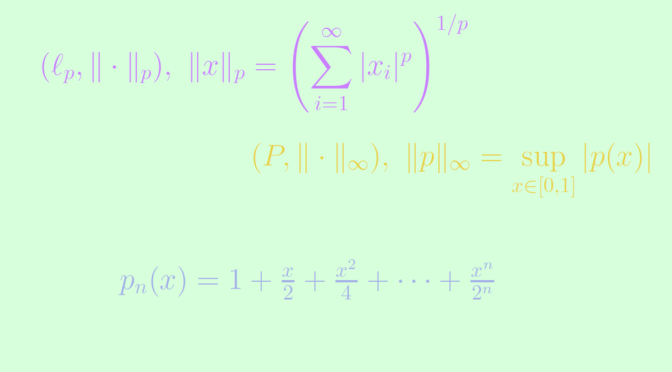# A non complete normed vector space

Consider a real normed vector space $$V$$. $$V$$ is called complete if every Cauchy sequence in $$V$$ converges in $$V$$. A complete normed vector space is also called a Banach space.

A finite dimensional vector space is complete. This is a consequence of a theorem stating that all norms on finite dimensional vector spaces are equivalent.

There are many examples of Banach spaces with infinite dimension like $$(\ell_p, \Vert \cdot \Vert_p)$$ the space of real sequences endowed with the norm $$\displaystyle \Vert x \Vert_p = \left( \sum_{i=1}^\infty \vert x_i \vert^p \right)^{1/p}$$ for $$p \ge 1$$, the space $$(C(X), \Vert \cdot \Vert)$$ of real continuous functions on a compact Hausdorff space $$X$$ endowed with the norm $$\displaystyle \Vert f \Vert = \sup\limits_{x \in X} \vert f(x) \vert$$ or the Lebesgue space $$(L^1(\mathbb R), \Vert \cdot \Vert_1)$$ of Lebesgue real integrable functions endowed with the norm $$\displaystyle \Vert f \Vert = \int_{\mathbb R} \vert f(x) \vert \ dx$$.

Let’s give an example of a non complete normed vector space. Let $$(P, \Vert \cdot \Vert_\infty)$$ be the normed vector space of real polynomials endowed with the norm $$\displaystyle \Vert p \Vert_\infty = \sup\limits_{x \in [0,1]} \vert p(x) \vert$$. Consider the sequence of polynomials $$(p_n)$$ defined by
$p_n(x) = 1 + \frac{x}{2} + \frac{x^2}{4} + \cdots + \frac{x^n}{2^n} = \sum_{k=0}^{n} \frac{x^k}{2^k}.$ For $$m < n$$ and $$x \in [0,1]$$, we have $\vert p_n(x) - p_m(x) \vert = \left\vert \sum_{i=m+1}^n \frac{x^i}{2^i} \right\vert \le \sum_{i=m+1}^n \frac{1}{2^i} \le \frac{1}{2^m}$ which proves that $$(p_n)$$ is a Cauchy sequence. Also for $$x \in [0,1]$$ $\lim\limits_{n \to \infty} p_n(x) = p(x) \text{ where } p(x) = \frac{1}{1 - \frac{x}{2}}.$ As uniform converge implies pointwise convergence, if $$(p_n)$$ was convergent in $$P$$, it would be towards $$p$$. But $$p$$ is not a polynomial function as none of its $$n$$th-derivative always vanishes. Hence $$(p_n)$$ is a Cauchy sequence that doesn't converge in $$(P, \Vert \cdot \Vert_\infty)$$, proving as desired that this normed vector space is not complete. More generally, a normed vector space with countable dimension is never complete. This can be proven using Baire category theorem which states that a non-empty complete metric space is not the countable union of nowhere-dense closed sets.